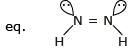Courses

# Test: Isomerism- 2

## 30 Questions MCQ Test Chemistry for JEE | Test: Isomerism- 2

Description
This mock test of Test: Isomerism- 2 for Class 11 helps you for every Class 11 entrance exam. This contains 30 Multiple Choice Questions for Class 11 Test: Isomerism- 2 (mcq) to study with solutions a complete question bank. The solved questions answers in this Test: Isomerism- 2 quiz give you a good mix of easy questions and tough questions. Class 11 students definitely take this Test: Isomerism- 2 exercise for a better result in the exam. You can find other Test: Isomerism- 2 extra questions, long questions & short questions for Class 11 on EduRev as well by searching above.
QUESTION: 1

### The compounds C2H5OC2H5 and CH3OCH2CH2CH3 are

Solution: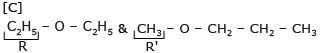R & R`  are different so these are metamers

QUESTION: 2

### C7H7Cl shows how many benzenoid aromatic isomers?

Solution:

[A] C7H7Cl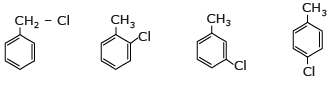QUESTION: 3

### How many minimum no. of C-atoms are required for position & geometrical isomerism in alkene?

Solution:

For positional isomerism in alkene 4C-atom required →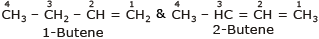For geometrical isomerism in alkene also 4c–atom required →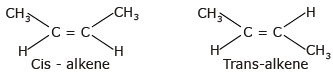QUESTION: 4

How many structural formula are possible when one of the hydrogen is replaced by a chlorine atom in anthracene?

Solution: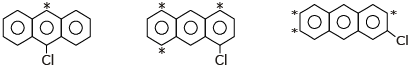QUESTION: 5

The principle involved in paper chromatography is

Solution:
QUESTION: 6

The number of cis-trans isomer possible for the following compound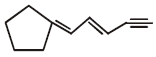Solution: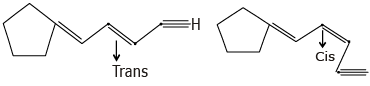QUESTION: 7

The number of isomers of dibromoderivative of an alkene (molear mass 186 g mol-1) is

Solution: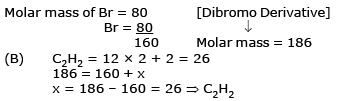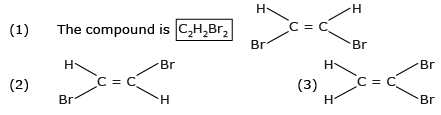QUESTION: 8

Increasing order of stability among the three main conformation (i.e. eclipse, anti, gauche) of ethylene glycol is:

Solution: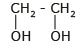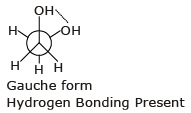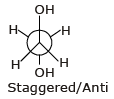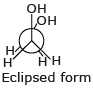Stability order ⇒ Gauche > Anti > Eclipse

QUESTION: 9

How many primary amines are possible for the formula C4H11N?

Solution:

C4H11N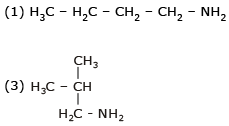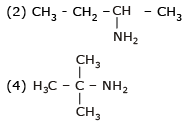QUESTION: 10

In which of the following compounds the carbon marked with asterisk is expected to have greatest positive charge?

Solution:
QUESTION: 11

How many planes (pos) are presents in Anthracene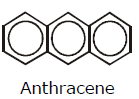Solution: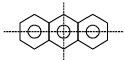(1) Vertical plane (2) Horizontal plane (3) Molecular Plane

QUESTION: 12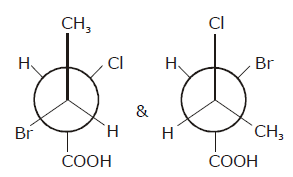Solution: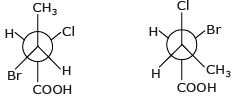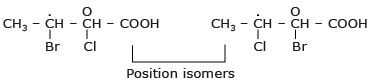QUESTION: 13

Geometrical isomers can be

Solution:

Geometrical Isomers can be Diastereomers or Enantiomers.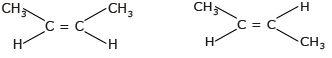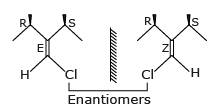Because geometrical isomers are around restricted rotation which can not be rotate or interconvertable.

QUESTION: 14

Phenol and benzyl alcohol are

Solution:
QUESTION: 15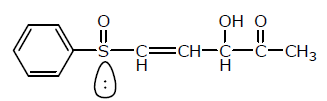Number of chiral centers are:

Solution: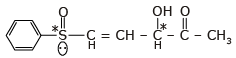Number of chiral centers = 2

QUESTION: 16

Which of the follownig compounds is (S)-4-chloro-1-methylcyclohexene ?

Solution:

(B) & (C) compounds are R-configuration.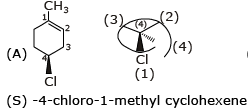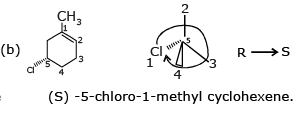QUESTION: 17

Which of the following compounds has two stereogenic centers (asymmetric carbons) ?

Solution: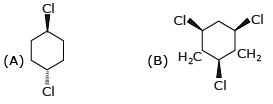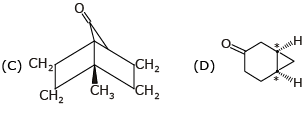QUESTION: 18

Which of the following structures represents a chiral compound ?

Solution: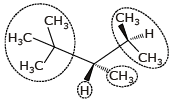All four substituent are different so it is chiral compound.

QUESTION: 19

Examine the compound on the right. How many stereoisomers having this constitution are possible ?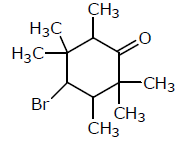Solution: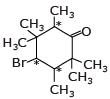Asymmetric = 23 = 8

QUESTION: 20

Which of the following heptanols are chiral 1-heptanol, 2-heptanol, 3-heptanol, 4-heptanol.

Solution: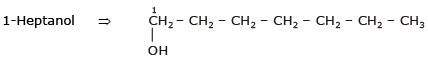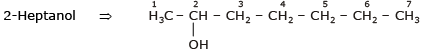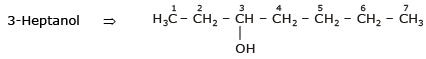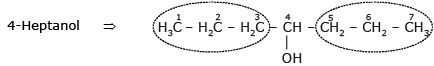1-Heptanol & 4-Heptanol are not chiral
2-Heptanol & 3-Heptanol are chiral compound.

QUESTION: 21

Which of the following will exhibit geometrical isomerism ?

Solution: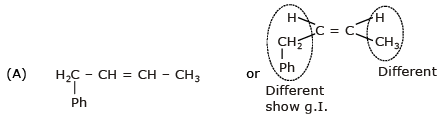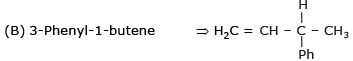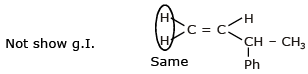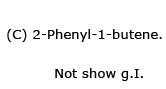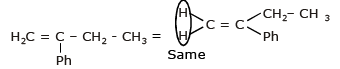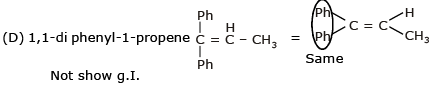QUESTION: 22

(A)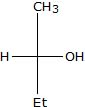(B)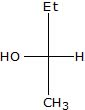Relation between given pair is

Solution: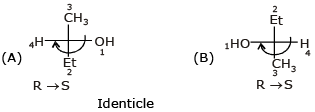QUESTION: 23

Following eclipsed form of propane is repeated after rotation of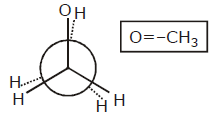Solution: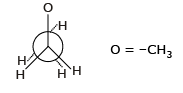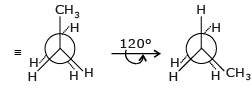QUESTION: 24

Stereoisomers differ from each other in what respect ?

Solution:

Stereoisomer differ from each other in configuration.

QUESTION: 25

The number of isomers of C5H10 is

Solution: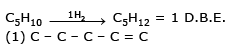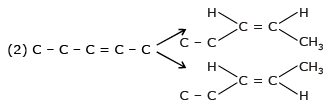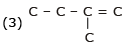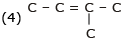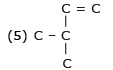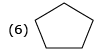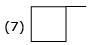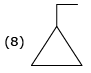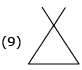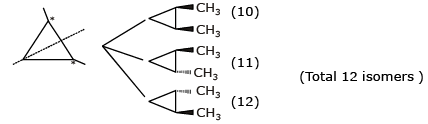QUESTION: 26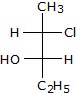The compound with the above configuration is called.

Solution: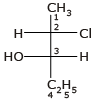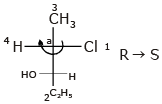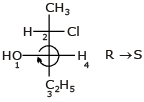(2s, 3s) -2-chloro-3-Hydroxy Pentane

QUESTION: 27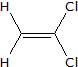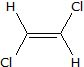The above compounds differ in

Solution: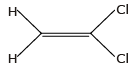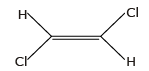The above compounds are different in structure.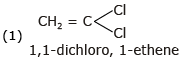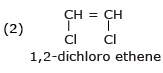QUESTION: 28

The compound C2H5OC2H5 and CH3OCH2CH2CH3 are

Solution: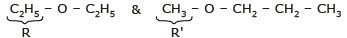R & R' are different so they are metamers.

QUESTION: 29

Which conformer of cyclohexane is chiral

Solution:

Twisted Boat form is chiral & optically active.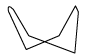QUESTION: 30

Minimum C atoms required for a compound to show geometrical isomerism :

Solution:

Any compound which show g.I. no C atom required.